# Algebra 1 : How to find the percent of increase

## Example Questions

### Example Question #71 : How To Find The Percent Of Increase

Find the percent of increase fromto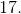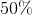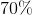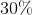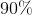Explanation:

For this type of problem we use this formula: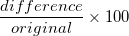"Difference" is simply the difference between the two numbers given, and "original" is the number that is stated usually after the word "from" , example: from ____ to ____. In this problem our formula will be filled in as follows: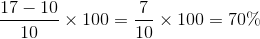This our percent increase.

### Example Question #72 : How To Find The Percent Of Increase

What is the percent increase fromto?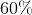Explanation:

For this type of problem we use this formula:"Difference" is simply the difference between the two numbers given, and "original" is the number that is stated usually after the word "from" , example: from ____ to ____. In this problem our formula will be filled in as follows: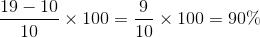This our percent increase.

### Example Question #73 : How To Find The Percent Of Increase

Find the percent increase fromto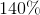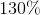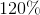Explanation:

For this type of problem we use this formula:"Difference" is simply the difference between the two numbers given, and "original" is the number that is stated usually after the word "from" , example: from ____ to ____. In this problem our formula will be filled in as follows: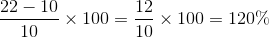This our percent increase.

### Example Question #74 : How To Find The Percent Of Increase

What is the percent increase fromto?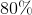Explanation:

For this type of problem we use this formula:"Difference" is simply the difference between the two numbers given, and "original" is the number that is stated usually after the word "from" , example: from ____ to ____. In this problem our formula will be filled in as follows: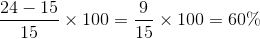This our percent increase.

### Example Question #75 : How To Find The Percent Of Increase

What is the percent increase fromto?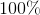Explanation:

For this type of problem we use this formula:"Difference" is simply the difference between the two numbers given, and "original" is the number that is stated usually after the word "from" , example: from ____ to ____. In this problem our formula will be filled in as follows: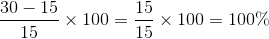This our percent increase.

### Example Question #76 : How To Find The Percent Of Increase

Find the percent increase fromto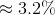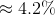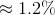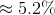Explanation:

For this type of problem we use this formula:"Difference" is simply the difference between the two numbers given, and "original" is the number that is stated usually after the word "from" , example: from ____ to ____. In this problem our formula will be filled in as follows: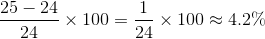This our percent increase.

### Example Question #77 : How To Find The Percent Of Increase

Find the percent increase fromto.Explanation:

For this type of problem we use this formula:"Difference" is simply the difference between the two numbers given, and "original" is the number that is stated usually after the word "from" , example: from ____ to ____. In this problem our formula will be filled in as follows:This our percent increase.

### Example Question #71 : Percent Of Change

What is the percent increase fromto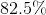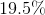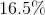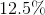Explanation:

For this type of problem we use this formula:"Difference" is simply the difference between the two numbers given, and "original" is the number that is stated usually after the word "from" , example: from ____ to ____. In this problem our formula will be filled in as follows: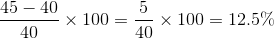This our percent increase.

### Example Question #78 : How To Find The Percent Of Increase

Find the percent increase fromto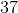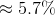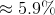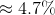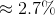Explanation:

For this type of problem we use this formula:"Difference" is simply the difference between the two numbers given, and "original" is the number that is stated usually after the word "from" , example: from ____ to ____. In this problem our formula will be filled in as follows: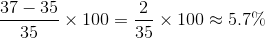This our percent increase.

### Example Question #79 : How To Find The Percent Of Increase

Find the percent increaseto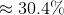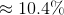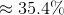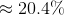Explanation:

For this type of problem we use this formula:"Difference" is simply the difference between the two numbers given, and "original" is the number that is stated usually after the word "from" , example: from ____ to ____. In this problem our formula will be filled in as follows: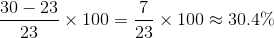This our percent increase.

### All Algebra 1 Resources